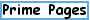Reference Database (references for the Prime Pages)Home
Search Site

Largest
Finding
How Many?
MersenneGlossary

Prime Curios!
e-mail list

FAQ
Prime Lists
Titans

Submit primes
This is the Prime Pages' interface to our BibTeX database.  Rather than being an exhaustive database, it just lists the references we cite on these pages.  Please let me know of any errors you notice.
References: [ Home | Author index | Key index | Search ]

#### Item(s) in original BibTeX format

@article{CDP97,
author={R. Crandall and K. Dilcher and C. Pomerance},
title={A Search for {Wieferich} and {Wilson} Primes},
abstract={An odd prime $p$ is called a {\it Wieferich prime} if $$2^{p-1} \equiv 1 \pmod{p^2};$$ alternatively, a {\it Wilson prime} if $$(p-1)!\equiv -1 \pmod{p^2}.$$ To date the only known Wieferich primes are $p=1093$ and
$3511$, while the only known Wilson primes are $p=5, 13$, and $563$. We
report that there exist no new Wieferich primes $p\lt 4\times 10^12$, and
no new Wilson primes $p\lt 5\times 10^8$. It is elementary that both defining
congruences above hold merely $\pmod{p}$, and it is sometimes estimated
on heuristic grounds that the ''probability'' that $p$ is Wieferich (independently:
that $p$ is Wilson) is about $1/p$. We provide some statistical data relevant
to occurrences of small values of the pertinent Fermat and Wilson quotients
$\pmod{p}$.},
journal= mc,
volume= 66,
year= 1997,
pages={433--449},
number= 217 ,
mrnumber={97c:11004}
}Another prime page by Reginald McLean